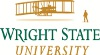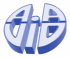Welcome to CS7200 - Algorithm Design and AnalysisThis course introduces concepts related to the design and analysis of algorithms. Specifically, it discusses recurrence relations, and illustrates their role in asymptotic and probabilistic analysis of algorithms. It covers in detail greedy strategies, divide and conquer techniques, dynamic programming and max flow - min cut theory for designing algorithms, and illustrates them using a number of well-known problems and applications. It also covers popular graph and matching algorithms, and basics of randomized algorithms and computational complexity. However, the depth of coverage of complexity classes and intractability, approximation algorithms, and randomized algorithms, will be as time permits. The programming assignments can be coded in Python or Java.

Assignments

Introductary slides

Stable Matching

Algorithm Analysis

Graphs

Greedy Algorithms I

Greedy Algorithms II

Demo of Dijkstra's algorithm

Slides for Huffman code, courtesy of Dan Melamad

Divide and Conquer I

Slides for Recurrence Relations, courtesy of Eric Torngs

Divide and Conquer II

Slides for Quicksort Randomization, courtesy of Norbert Zeh

Slides for Quicksort, courtesy of Charles Leiserson

Slides for Selection, courtesy of Daniel Gildea

Slides for Probabilistic Analysis, courtesy of Keith Schwarz

Dynamic Programming I

Dynamic Programming II

Network Flow I

Interactive demo of Ford-Fulkerson algorithm

Network Flow II

Network Flow III

Intractability I

Intractability II

P-Space

Extending Tractability

Approximation Algorithms

K-means clustering algorithm

Local Search

Randomized Algorithms